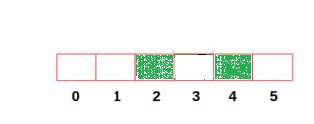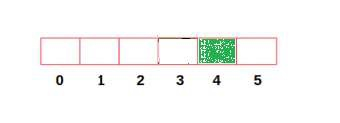# Find maximum distance between any city and station in C++

## Concept

With respect of the given number of cities N numbered from 0 to N-1 and the cities in which stations are located, our task is to determine the maximum distance between any city and its nearest station. It should be noted that the cities with stations can be given in any order.

## Input

numOfCities = 6, stations = [2, 4]

## Output

2

## Input

numOfCities = 6, stations = 

## Output

4
• The following figure indicates the first example containing 6 cities and the cities with stations highlighted with green color. So, in this case, the farthestcities from its nearest stations are 0 at a distance of 2. Hence maximum distance is 1.• In the second example, the farthest city from its nearest station is 0 which is at a distance of 4. Hence maximum distance is 4.## Method

Here, there are three possible cases in this problem −

• First case indicates when the farthest city is between two stations.

• Second case indicates when the farthest city is on the left side of the first station.

• Last case indicates when the farthest city is on the right side of the last station.

Following algorithm is implemented to solve the above problem −

• We initialize a boolean array of size N (number of cities) with False. After that mark the values of cities with stations as True

• Next we initialize a variable dist with 0. We have to initialize another variablemaxDist with value which is same to the first city with station (used for second case).

• Begin looping through all the cities ony by one.

• It has been observed that if the current city has a station, and then assign the maximum of (dist+1)//2 and maxDist to maxDist (used for first case). In addition,assign 0 to dist.

• Else, increment dist.

• Finally, return the maximum of dist and maxDist (used for third case).

## Example

Live Demo

// C++ program to calculate the maximum
// distance between any city
// and its nearest station
#include<bits/stdc++.h>
using namespace std;
// Shows function to compute the maximum
// distance between any city and its nearest station
int findMaxDistance(int numOfCities1,int station1[],int N){
// Used to initialize boolean list
bool hasStation[numOfCities1 + 1] = {false};
// Used to assign True to cities containing station
for (int city1 = 0; city1 < N; city1++){
hasStation[station1[city1]] = true;
}
int dist1 = 0;
int maxDist1 = INT_MAX;
for(int i = 0; i < N; i++){
maxDist1 = min(station1[i],maxDist1);
}
for (int city1 = 0; city1 < numOfCities1; city1++){
if (hasStation[city1] == true){
maxDist1 = max((dist1 + 1) / 2, maxDist1);
dist1 = 0;
}
else
dist1 += 1;
}
return max(maxDist1, dist1);
}
//Driver code
int main(){
int numOfCities1 = 6;
int station1[] = {2,4};
int N = sizeof(station1)/sizeof(station1);
cout << "Max Distance:" << findMaxDistance(numOfCities1,
station1, N);
}

## Output

Max Distance:2